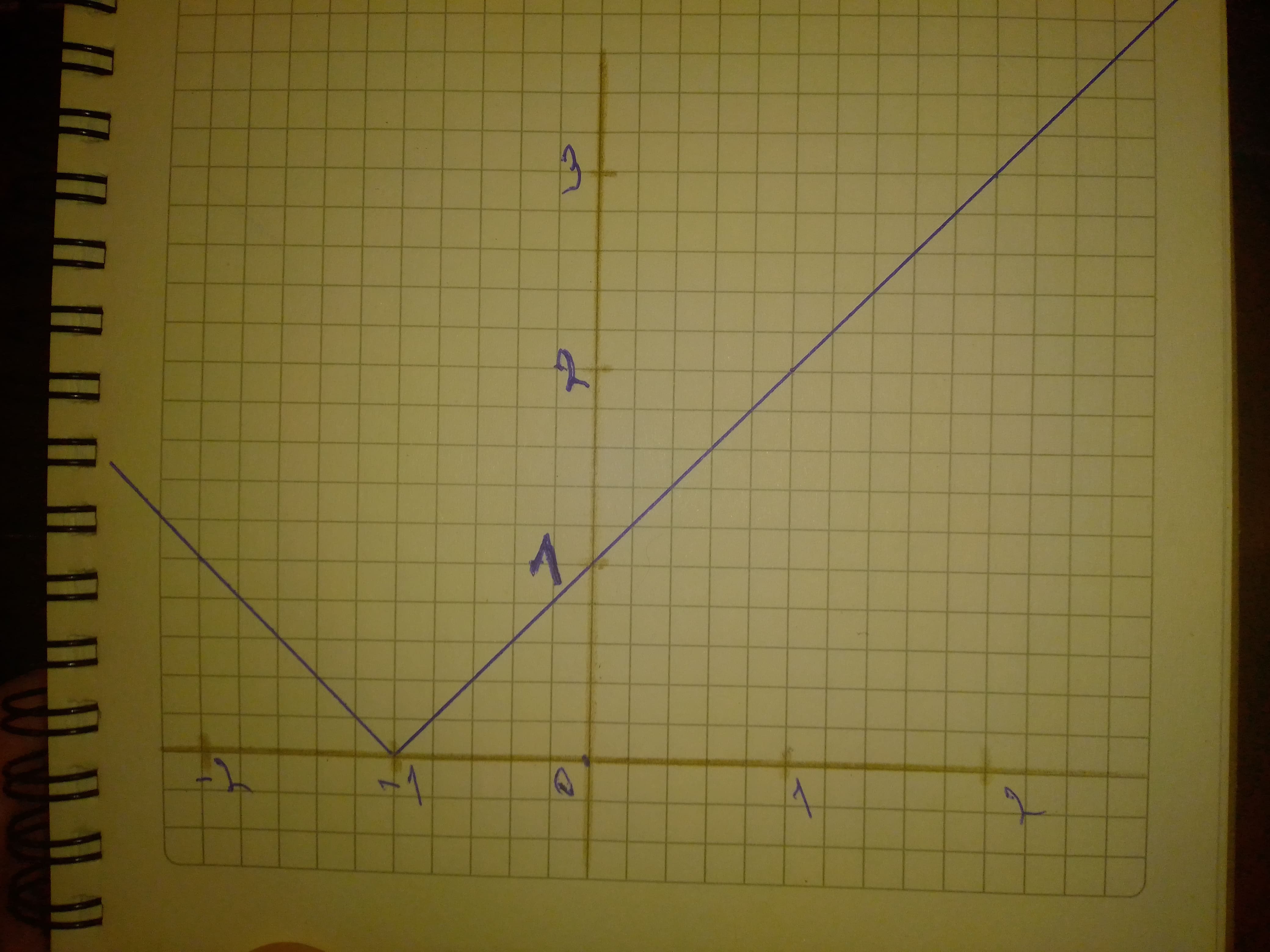# Sketch a graph of the function. Use transformations of functions when ever possible. f(x)=|x+1|beljuA 2020-10-20 Answered
Sketch a graph of the function. Use transformations of functions when ever possible.
$$\displaystyle{f{{\left({x}\right)}}}={\left|{x}+{1}\right|}$$

• Questions are typically answered in as fast as 30 minutes

### Plainmath recommends

• Get a detailed answer even on the hardest topics.
• Ask an expert for a step-by-step guidance to learn to do it yourself.davonliefI

Step 1
Shown is the graph of $$\displaystyle{f{{\left({x}\right)}}}={\left|{x}+{1}\right|}$$
x is on the horizontal axis and y is on the vertical axis
Step 2